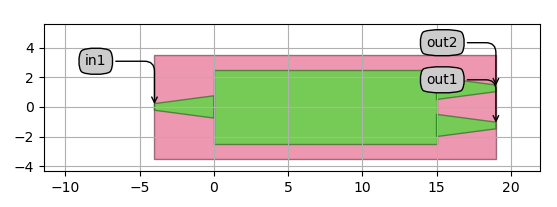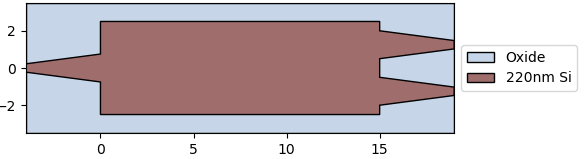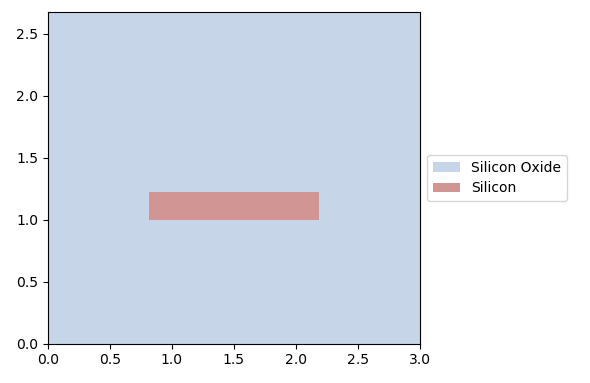# MMI PCell¶

In this session, you will learn how to design a multi-mode interferometer (MMI). This example serves as a starting point to design more complex components and circuits. The MMI we are going to show is part of SiFab, the demonstration library included with this training material.## Define the PCell¶

In order to design the MMI, we define a Python class derived from i3.PCell and we call it MMI1x2. Inside this class we define another class, class Layout, derived from i3.LayoutView. This class will contain all the geometric information of the MMI.

class MMI1x2(i3.PCell):
""" MMI with 1 input and 2 outputs.
"""
_name_prefix = "MMI1x2"
trace_template = i3.TraceTemplateProperty(default=SiWireWaveguideTemplate(),
doc="Trace template of the access waveguide")
width = i3.PositiveNumberProperty(default=4.0, doc="Width of the MMI section.")
length = i3.PositiveNumberProperty(default=20.0, doc="Length of the MMI secion.")
taper_width = i3.PositiveNumberProperty(default=1.0, doc="Width of the taper.")
taper_length = i3.PositiveNumberProperty(default=1.0, doc="Length of the taper")
waveguide_spacing = i3.PositiveNumberProperty(default=2.0, doc="Spacing between the waveguides.")

def validate_properties(self):
if self.width < self.waveguide_spacing + self.taper_width:
raise i3.PropertyValidationError(self,
"The sum of waveguide_spacing and taper_width exceeds the width of "
"the MMI",
{"width": self.width,
"waveguide_spacing": self.waveguide_spacing,
"taper_width": self.taper_width}
)
if self.waveguide_spacing < self.taper_width:
raise i3.PropertyValidationError(self,
"The waveguides will overlap: waveguide_spacing is too small or width "
"is too big",
{"taper_width": self.taper_width,
"waveguide_spacing": self.waveguide_spacing}
)
return True

class Layout(i3.LayoutView):
def _generate_elements(self, elems):
length = self.length
width = self.width
taper_length = self.taper_length
taper_width = self.taper_width
half_waveguide_spacing = 0.5 * self.waveguide_spacing
core_layer = self.trace_template.core_layer
core_width = self.trace_template.core_width
# Si core
elems += i3.Rectangle(layer=core_layer,
center=(0.5 * length, 0.0),
box_size=(length, width))
elems += i3.Wedge(layer=core_layer,
begin_coord=(-taper_length, 0.0),
end_coord=(0.0, 0.0),
begin_width=core_width,
end_width=taper_width)
elems += i3.Wedge(layer=core_layer,
begin_coord=(length, half_waveguide_spacing),
end_coord=(length + taper_length, half_waveguide_spacing),
begin_width=taper_width,
end_width=core_width)
elems += i3.Wedge(layer=core_layer,
begin_coord=(length, -half_waveguide_spacing),
end_coord=(length + taper_length, -half_waveguide_spacing),
begin_width=taper_width,
end_width=core_width)
center=(0.5 * length, 0.0),
box_size=(length + 2 * taper_length, width + 2.0))
return elems

def _generate_ports(self, ports):
length = self.length
taper_length = self.taper_length
trace_template = self.trace_template
half_waveguide_spacing = 0.5 * self.waveguide_spacing

ports += i3.OpticalPort(name="in1", position=(-taper_length, 0.0),
angle=180.0, trace_template=trace_template)
ports += i3.OpticalPort(name="out1",
position=(length + taper_length, -half_waveguide_spacing),
angle=0.0, trace_template=trace_template)
ports += i3.OpticalPort(name="out2",
position=(length + taper_length, half_waveguide_spacing),
angle=0.0, trace_template=trace_template)
return ports



Let’s analyze the code above:

1. Properties. We defined a list of properties that will be used to design the MMI. Five properties are defined using i3.PositiveNumberProperty and one is defined using i3.TraceTemplateProperty. The order of these properties can be altered. Each property has a default value: if the user does not supply a value, this default will be used.

2. Properties validation. We defined a validate_properties method. This method is used to check that the layout is self-consistent. It will raise an error when the sum of the waveguide spacing and the taper width exceeds the MMI width, or when the waveguide spacing is smaller than the taper width. This way, we can ensure that we don’t make mistakes when we instantiate the layout and assign values to the MMI properties.

3. Layout class. We defined the Layout class inside the MMI1x2 PCell. This class is a Layout View. IPKISS can attach many different views to a PCell, e.g. layout, simulation, cross-sections, etc.

1. Inside the method _generate_elements, we added five geometric elements:

• 1 Rectangle, drawn on the core layer of our trace template (self.trace_template.core_layer), representing the multi-mode section of the MMI;
• 3 Wedges, drawn on the core layer of our trace template (self.trace_template.core_layer), representing the tapers at the input and output;
• 1 Rectangle, drawn on the cladding layer of our trace template (self.trace_template.cladding_layer), representing the cladding.

These five geometric elements are drawn using the properties defined at the beginning of the class MMI1x2. For example, the length property is used by addressing it as self.length. Calculations of the dimension and position of the elements are performed with standard Python functions.

2. Ports are added in the method _generate_ports. Each port is created using i3.OpticalPort and needs the following properties to be set:

• name: name of the port.
• position: position of the port.
• angle: direction a waveguide leaving the port has to go to. 0 degrees is parallel to the x-axis, going towards east.
• trace_template: it denotes the trace template of waveguide used at the port.

The trace template used is set at the PCell level and is passed on as a property as self.trace_template.

## Visualize the layout¶

To visualize the layout, we first instantiate an object using the PCell MMI1x2 and then we instantiate the Layout class of this object. When the MMI object is instantiated, the value of its properties can be modified, as shown below.

mmi = pdk.MMI1x2(trace_template=pdk.SiWireWaveguideTemplate(),
width=5.0,
length=15.0,
taper_width=1.5,
taper_length=4.0,
waveguide_spacing=2.5)
mmi_layout = mmi.Layout()


We can now visualize the layout and export it to GDSII:

mmi_layout.visualize(annotate=True)
mmi_layout.write_gdsii("first_mmi.gds")## Virtual fabrication and cross section¶

In IPKISS, in addition to the visualize function, there is also the option to run a virtual fabrication using the visualize_2d function. The output will show the actual fabricated layer stacks. In our case, we can see that the MMI is fabricated on 220 nm Si and is surrounded by an oxide cladding. We can also visualize the cross section at specific coordinates.

mmi_layout.visualize_2d()